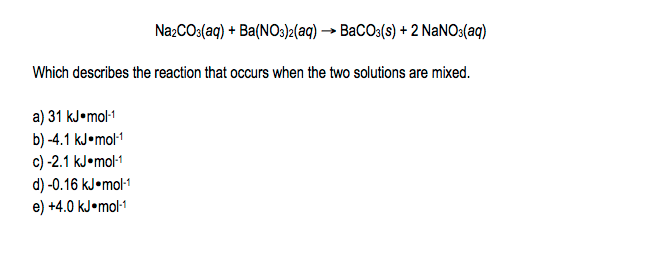# Problem: A 0.500-L sample of 0.400 M Na2CO3 (aq) is added to 0.500 L of 0.400 M Ba(NO 3)2 (aq) in a calorimeter with a total heat capacity equal to 656 J•K –1 at a constant pressure of one bar. The temperature change is +1.25 K. Use these data to calculate the value of ΔH°rxn for the equation:

###### FREE Expert Solution
90% (220 ratings)
###### Problem Details

A 0.500-L sample of 0.400 M Na2CO3 (aq) is added to 0.500 L of 0.400 M Ba(NO 3)2 (aq) in a calorimeter with a total heat capacity equal to 656 J•K –1 at a constant pressure of one bar. The temperature change is +1.25 K. Use these data to calculate the value of ΔH°rxn for the equation:Frequently Asked Questions

What scientific concept do you need to know in order to solve this problem?

Our tutors have indicated that to solve this problem you will need to apply the Thermochemical Equations concept. You can view video lessons to learn Thermochemical Equations. Or if you need more Thermochemical Equations practice, you can also practice Thermochemical Equations practice problems.

What is the difficulty of this problem?

Our tutors rated the difficulty ofA 0.500-L sample of 0.400 M Na2CO3 (aq) is added to 0.500 L ...as high difficulty.

How long does this problem take to solve?

Our expert Chemistry tutor, Jules took 5 minutes and 19 seconds to solve this problem. You can follow their steps in the video explanation above.